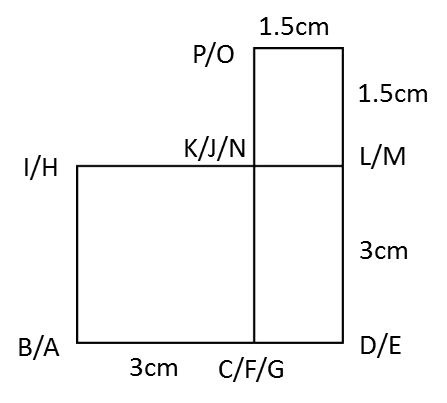# 10.3 SPM Practice (Long Questions)

Question 7 (12 marks):
You are not allowed to use graph paper to answer this question.

(a) Diagram 7.1 shows a solid prism with a rectangular base JKLM on a horizontal plane. The surface MQRSTL is the uniform cross section of the prism. Triangle STU and PQR are horizontal planes. Edges PJ, RS and UK are vertical. PQ = TU = 3 cm.Diagram 7.1

Draw to full scale, the elevation of the solid on a vertical plane parallel to KL as viewed from X.

(b)
Another solid cuboid with rectangle base JMDE is combined to the prism in Diagram 7.1 at the vertical plane JMQP. The composite solid is as shown in Diagram 7.2. The base EJKLMD lies on a horizontal plane.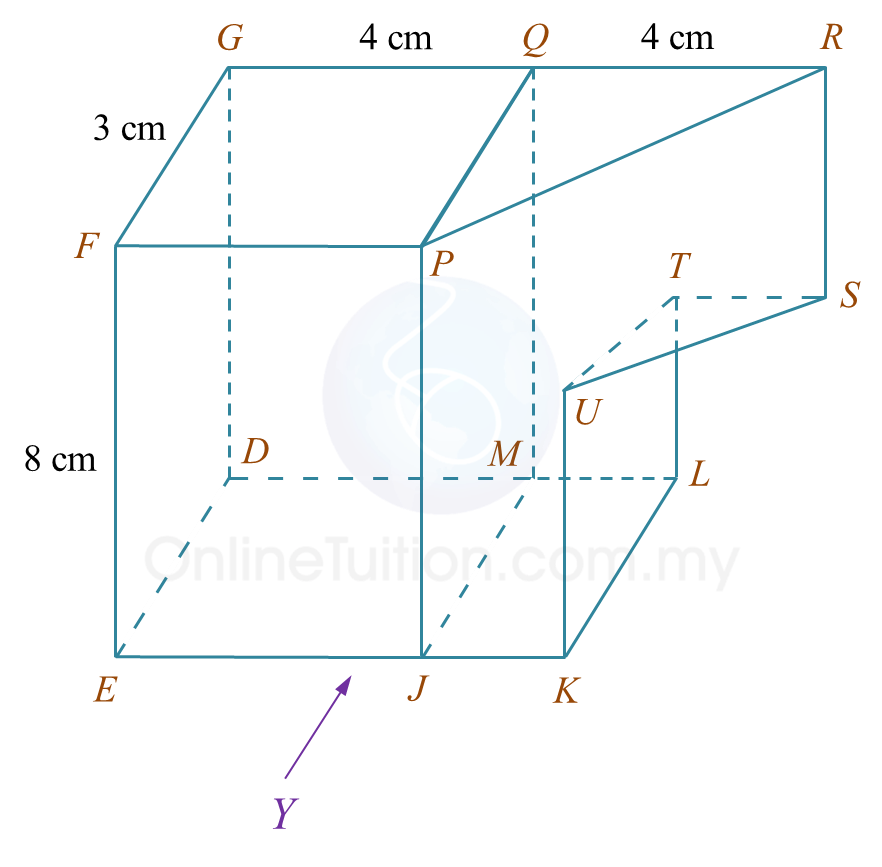Diagram 7.2

Draw to full scale,
(i) the elevation of the composite solid on a vertical plane parallel to EJK as viewed from Y.
(ii) the plan of the composite solid.

Solution:
(a)(b)(i)(b)(ii)# 10.3 SPM Practice (Long Questions)

Question 6 (12 marks):
You are not allowed to use graph paper to answer this question.

(a) Diagram 6.1 shows two solid right prisms joined at the vertical plane EGLK. The planes JKL and MNP are the uniform cross sections of the prism HEGLJK and prism EFGMNP respectively. The base EFGH is a rectangle which lies on a horizontal plane. Edges HJ and EK are vertical.Diagram 6.1

Draw, to full scale, the plan of the composite solid.

(b)
Another solid half cylinder with a diameter of 4 cm is joined to the prism in Diagram 9.1 at the vertical plane EFQR. The composite solid is as shown in Diagram 6.2. The base HETFG lies on a horizontal plane.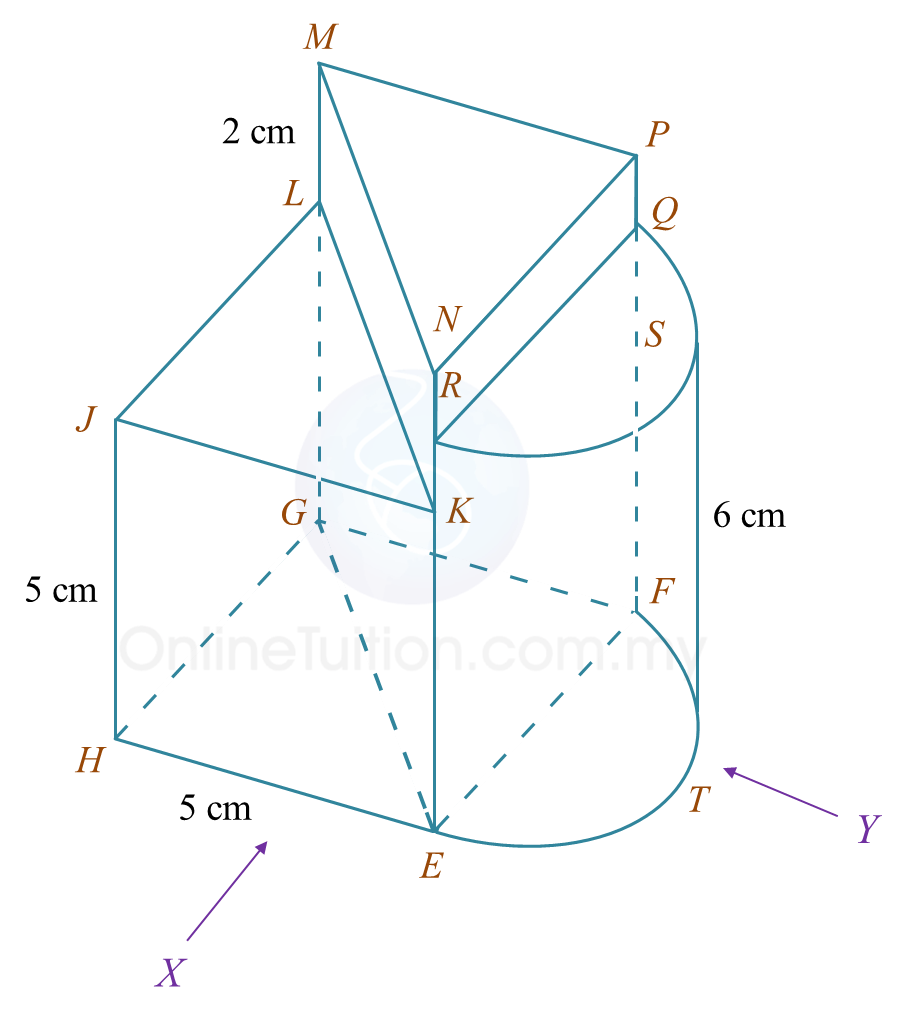Diagram 6.2

Draw to full scale,
(i) the elevation of the composite solid on a vertical plane parallel to HE as viewed from X.
(ii) the elevation of the composite solid on a vertical plane parallel to EF as viewed from Y.

Solution:
(a)(b)(i)(b)(ii)# 10.3 SPM Practice (Long Questions)

Question 5 (12 marks):
You are not allowed to use graph paper to answer this question.

(a)
Diagram 5.1 shows a pyramid with rectangular base ABCD on a horizontal plane. Vertex E is vertically above C. Triangles BCE and DCE are vertical planes. Triangles ABE and ADE are inclined planes.Diagram 5.1

Draw to full scale, the plan of the solid.

(b)
Another solid cuboid with rectangle base BLKC is joined to the pyramid in Diagram 5.1 at the vertical plane BCFM. The composite solid is as shown in Diagram 5.2. The base ABLKCD lies on a horizontal plane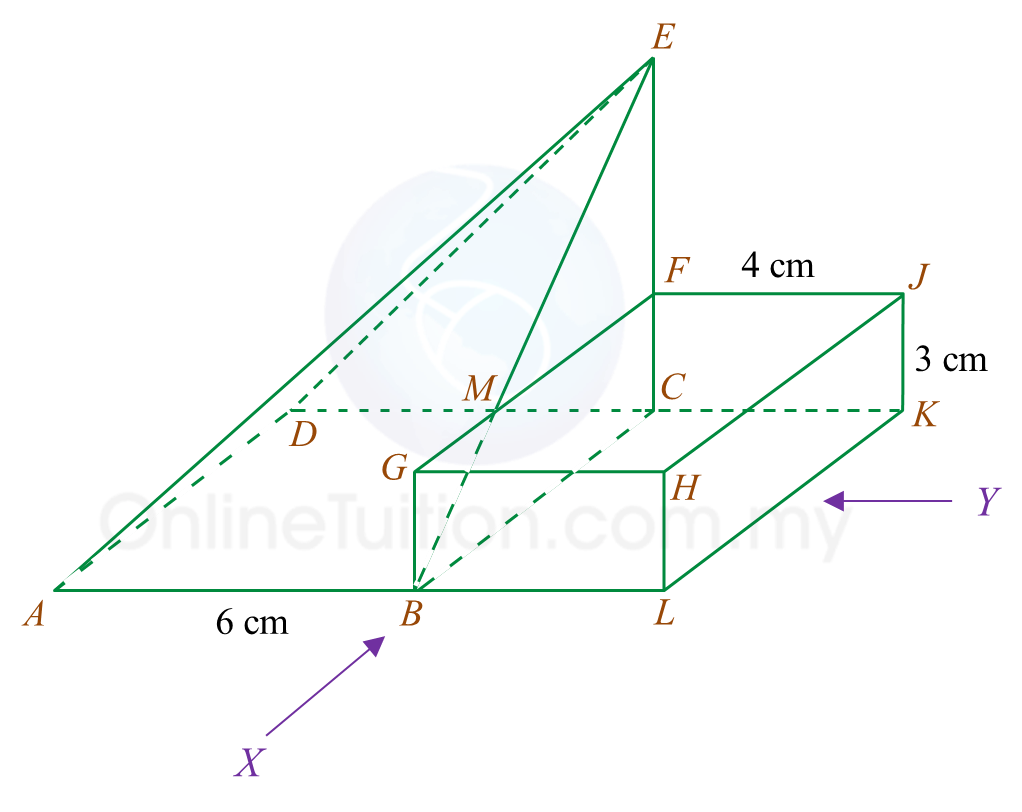Diagram 5.2

Draw to full scale,
(i) the elevation of the composite solid on a vertical plane parallel to ABL as viewed from X.

(ii)
the elevation of the composite solid on a vertical plane parallel to LK as viewed from Y.

Solution:

(a)(b)(i)(b)(ii)# 10.3 SPM Practice (Long Questions)

Question 4: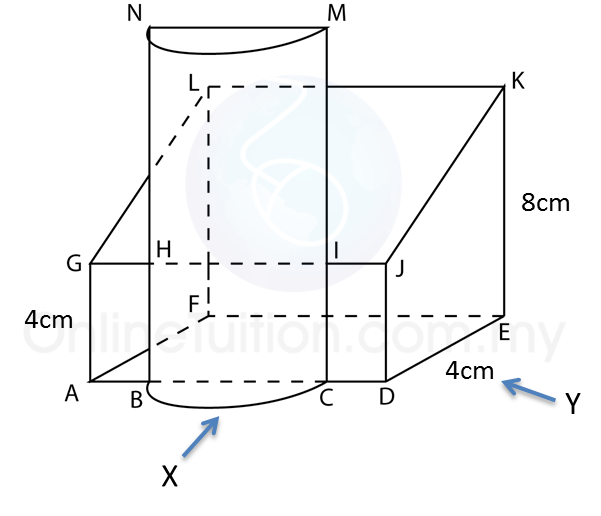The diagram above shows a solid consisting of a right prism and a half-cylinder which are joined at the plane HICB. The base ABCDEF is on a horizontal plane. The rectangle LKJG is an inclined plane. The vertical plane JDEK is the uniform cross-section of the prism. AB = CD = 2 cm. BC = 4 cm. CM = 12 cm.
Draw to full scale
(a)  The plan of the solid
(b) The elevation of the solid on a vertical plane parallel to ABCD as viewed from X.
(c)  The elevation of the solid on a vertical plane parallel to DE as viewed from Y.

Solution:
(a)(b)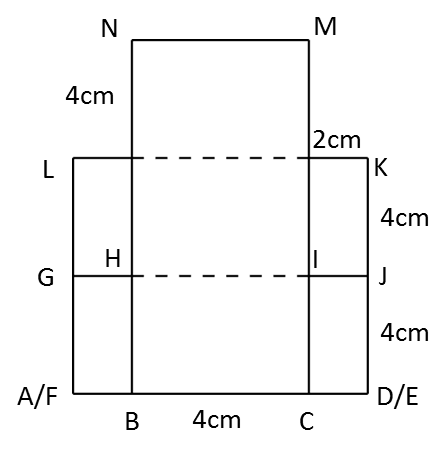(c)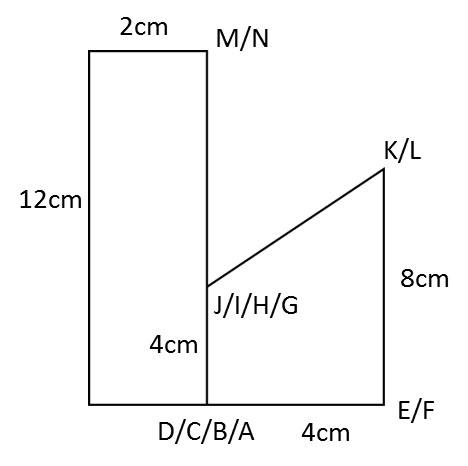# 10.3 SPM Practice (Long Questions)

Question 1: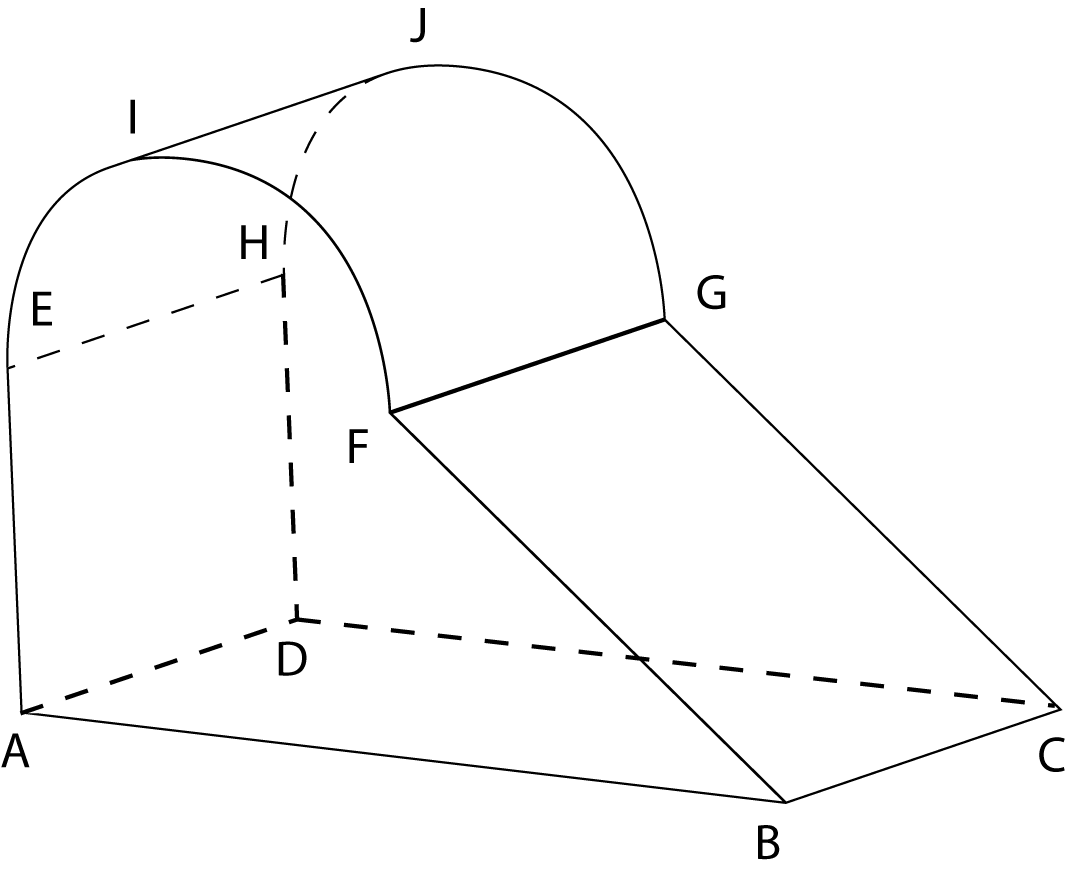The diagram above shows a solid consisting of a right prism and a half-cylinder which are joined at the plane EFGH. EF is the diameter of the semi-circle and is equal to 3 cm.

The base ABCD is on a horizontal plane and AB = 6 cm, BC = 4 cm. The vertical plane ABFE is the uniform cross-section of the prism.

Draw to full scale, the plan of the solid.

Solution:Question 2:Diagram above shows a solid right prism with rectangular base ABCD on a horizontal table. The vertical plane ABEHIL is the uniform cross-section of the prism. Rectangle LIJK, IHGJ and HEFG are inclined planes. AL, DK, BE and CF are vertical edges.

Given BC
= 4 cm, AB = 6cm. EB = FC = LA = KD = 4cm, The vertical height of  I and J from the rectangular base ABCD = 3cm, while the vertical height of H and G from the rectangular base ABCD = 5cm.

Draw to full scale, the elevation of the solid on a vertical plane parallel to BC as viewed from X.

Solution: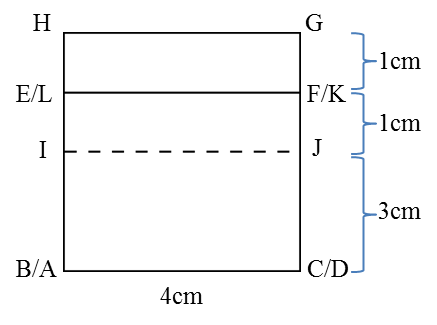# 10.2 Plans and Elevations

10.2 Plans and Elevations

1.  Plan is the image formed when solid is viewed from the top. Its orthogonal projection lies on the horizontal plane.
2.  Elevation is the image formed when a solid is viewed from the front or from the side. Its orthogonal projection lies on the vertical plane.
3.  In drawing plans and elevations of solids,
(a)  Visible edges should be drawn using solid lines(──),
(b)  Hidden edges whose views are blocked should be drawn using dashed lines (- - -).

Example:# 10.3 SPM Practice (Long Questions)

Question 3:The diagram above shows a right prism attach to a cuboid at one of its plane. ABCG and CDEF, IJNH and KLMJ are horizontal planes, ABIH, AGNH and GCJN are vertical planes while IBCJ is an inclined plane. HA = 3 cm, BC = 3 cm, CD = 1.5 cm, HI = NJ = JK = 3 cm. Draw to full scale

(a) The elevation of the solid on a vertical plane parallel to AB as viewed from X.(b) A solid semi-cylinder is joined to the solid in A as show in the diagram above. JPON is a vertical plane and JP = 1.5 cm. Draw to full scale
i. The plan of the solid
ii.   The elevation of the solid on a vertical plane parallel to BCD as viewed from Y.

Solution:

(a)(b)(i)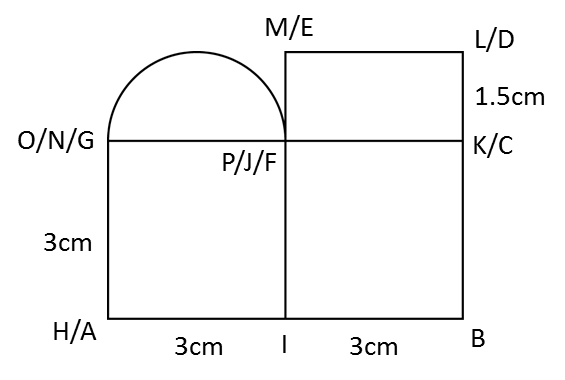(b)(ii)